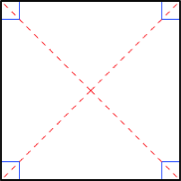## ASVAB Math Knowledge Practice Test 816018

Question 2 of 5
###### Rectangle & Square

A rectangle is a parallelogram containing four right angles. Opposite sides (a = c, b = d) are equal and the perimeter is the sum of the lengths of all sides (a + b + c + d) or, comonly, 2 x length x width. The area of a rectangle is length x width. A square is a rectangle with four equal length sides. The perimeter of a square is 4 x length of one side (4s) and the area is the length of one side squared (s2).If the area of this square is 25, what is the length of one of the diagonals?

 3$$\sqrt{2}$$ 8$$\sqrt{2}$$ 6$$\sqrt{2}$$ 5$$\sqrt{2}$$